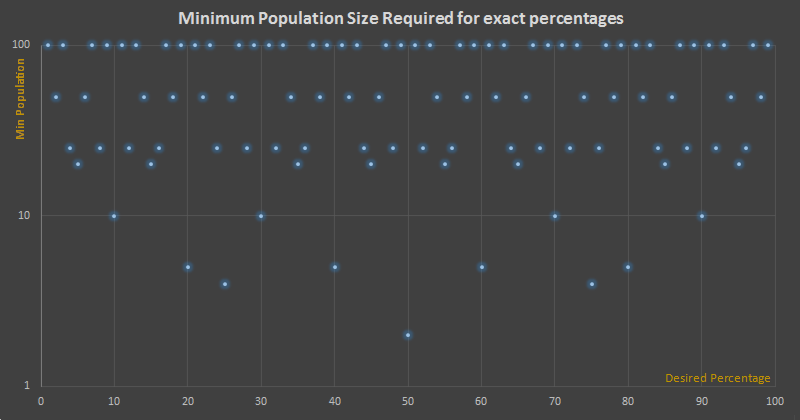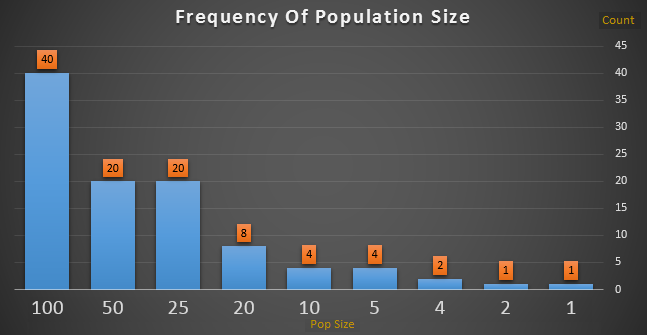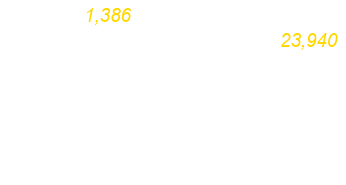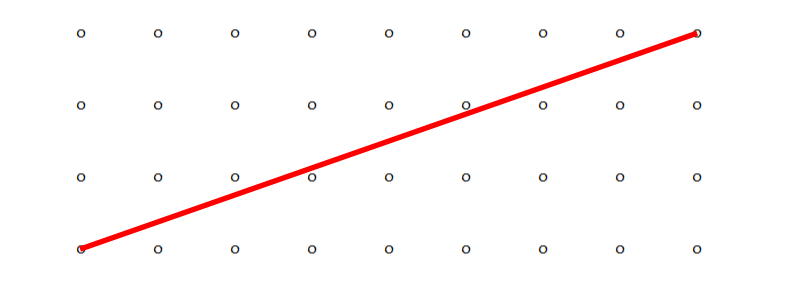﻿ Exact Percentages and Prime Factors# Exact Percentages and Prime Factors

 One of your friends is doing a survey to find out if people prefer coffee rather than tea. They tell you that exactly 75% of the people they surveyed prefer coffee, what is the smallest size sample of people that could have been questioned? The answer, of course, is four. If three out of four people prefer coffee that’s 75%. There are lots of other possible answers that result in 75%, for instance fifteen people out of twenty, plus of course seventy-five people out of a hundred (and even 750,000 out of a million).All of these answers have the ratio of 0.75, but 3/4 is the simplest ratio. Hold that thought, we’ll come back to it shortly, but first let’s take a little detour into the world of exact percentages. If we look at all the exact (integer) percentages from 1%-100%, what is the minimum number of people needed to be able to define each ratio? To get 1%, obviously, we need a denominator of 100, so to get exactly 1% the smallest sample size would be 100 people. To get 50% we need just two people, and to get 75% (and also 25%) we’d need four people.

Below is a graph showing the distribution of the smallest audience size needed to get an integer percentage.

The x-axis shows the desired percentage, and the y-axis shows the minimum audience size,
(I’ve used a log scale here as it makes things look a little prettier).It’s a very pretty pattern, and as you can see is symmetrical around the mid-point.

(A little imagination shows why this should be the case … if you have trouble with this, think back to the 25% and 75% examples that need a denominator of four and work from there).

The table below shows a histogram of the distribution of the sample sizes.Forty percentages require a denominator of 100 people to get an exact percentage, for instance 67%. This is because 67/100, as a fraction, cannot be simplified.

What this puzzle reduces down to is the simplification of fractions. A percentage is ratio of two terms.

### Simplifying Fractions

 To simplify a fraction we need to remove common factors from both the numerator and denominator Essentially - “By what factor can we divide both the top and bottom by?” Mathematically, to simplify a fraction as much as possible we need to divide the top and bottom by the Greatest Common Divisor (also known as the Greatest Common Factor). It is the largest possible integer that divides both numbers without any remainder. There are various ways to calculate this, but one of the more interesting is to break a number down into its prime factorsAll positive numbers (greater than one if you want to be mathematically pedantic), are either a prime number or can be written as a product of two or more prime numbers.

For instance the prime factors of 23,940 and 1,386 are:

23,940 = 2 × 2 × 3 × 3 × 5 × 7 × 19       can also be written as:      22.32.51.71.191

1,386 = 2 × 3 × 3 × 7 × 11       can also be written as:      21.32.71.111

We can calculate the Greatest Common Factor by observing all the prime factors that these two numbers have in common. This will allow us to simplify the fraction 1,386/23,940The prime factors in common are: 2 × 3 × 3 × 7 = 126, so we can divide both sides by 126 and be confident there will be no remainder.

Thus, we can simplify 1,386/23,940 into 11/190

There are no more factors in common between 11 and 190, so there is nothing left to divide by (in fact 11 is also prime, so by definition it has no factors other than one and itself!)

We've simplified the fraction as much as we can!

Sometimes pairs of numbers have no prime factors in common e.g. 525 (3 × 5 × 5 × 7) and 209 (11 × 19)

When numbers have no common factors (other than, trvially, one!) that are described as being relatively prime.

Ratios of relatively prime numbers cannot be simplified. Looping back to our exact percentage opening puzzle, all the percentages that require a minimum population size of a hundred people are audiences that are relatively prime to 100.

### Try it for yourselfPrime Factor Calculator Enter a number into the box below and press Calculate to determine its prime factors

### Relatively prime in pictures

Here's another little interesting thing, which is obvious now that you know about relative primes, but if you have a grid where the number of units in each dimension are relatively prime to each other, then a diagonal line drawn between opposite corners will not pass through any other point!You can find a complete list of all the articles here.Click here to receive email alerts on new articles.